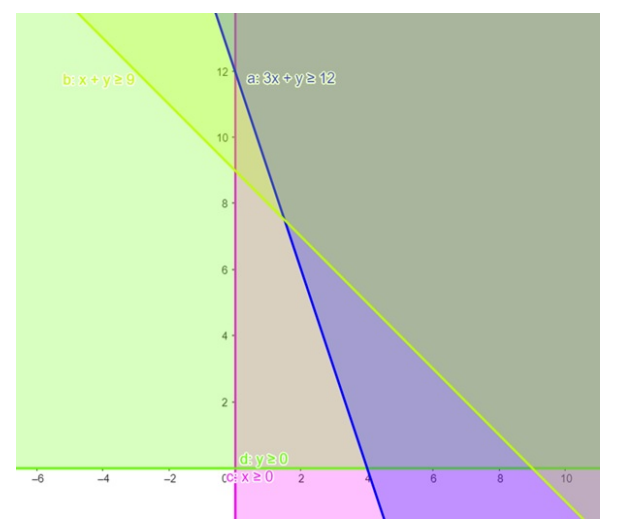# Solve the given inequalities

Question:

Solve the given inequalities $3 x+y \geq 12, x+y \geq 9, x \geq 0, y \geq 0$.graphically in two - dimensional plane.

Solution:

The graphical representation of $3 x+y \geq 12, x+y \geq 9$

$x \geq 0, y \geq 0$ is given by common region in the figure below.

$3 x+y \geq 12 \ldots \ldots$ (1)

$x+y \geq 9 \ldots \ldots$ (2)

$x \geq 0 \ldots \ldots$ (3)

$y \geq 0 \ldots \ldots$ (4)

Inequality (1) represents the region above line $3 x+y=12$ (including the line $3 x+y=12$ ).

Inequality (2) represents the region above line $x+y=9$ (including the line $x+y=9$ ).

Inequality (3) represents the region in front of line $x=0$ (including the line $x=0$ ).

Inequality (4) represents the region above line $y=0$ (including the line $y=0$ )

It is clear from the graph, that the region is unbounded.

Therefore, the following system of inequation is an unbounded set.

This can be represented as follows,